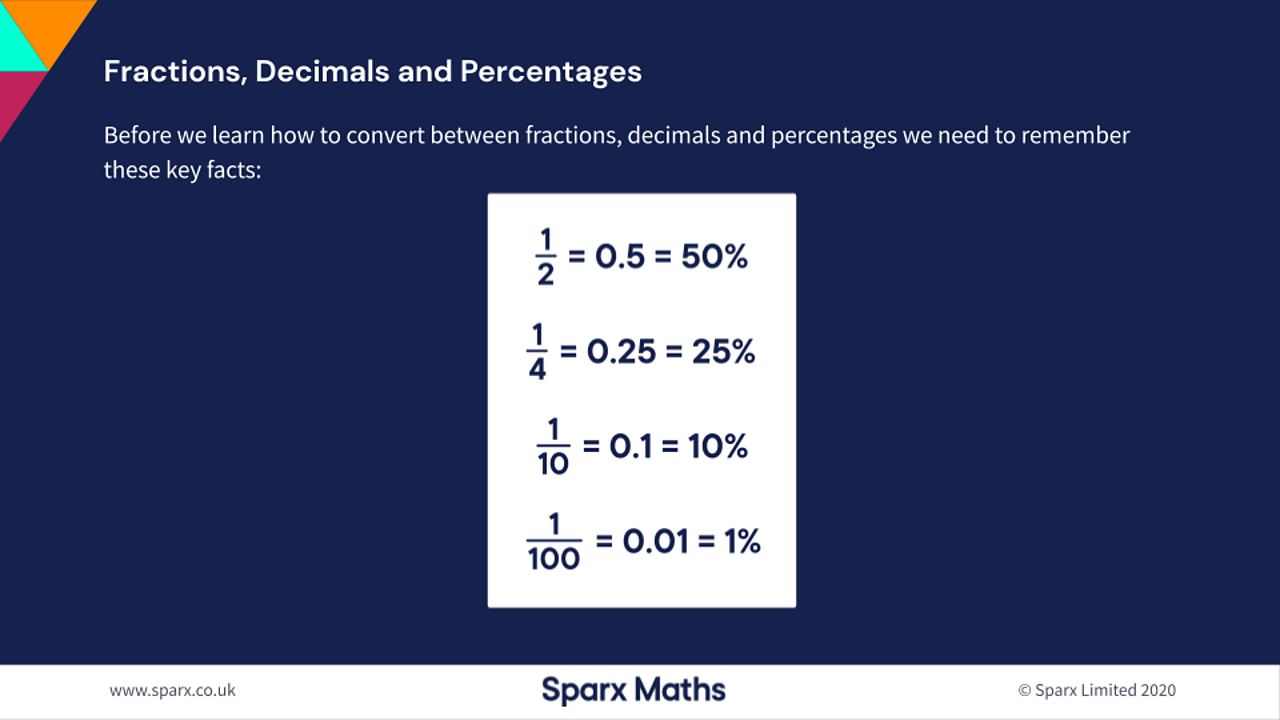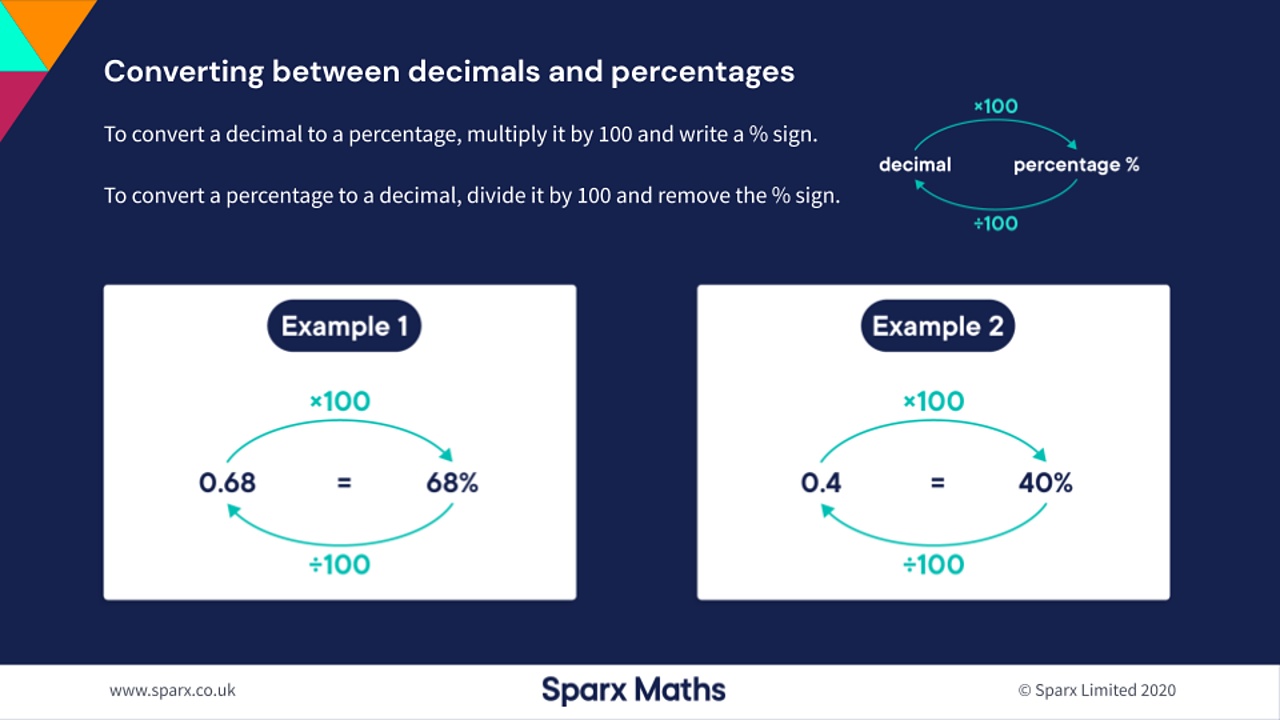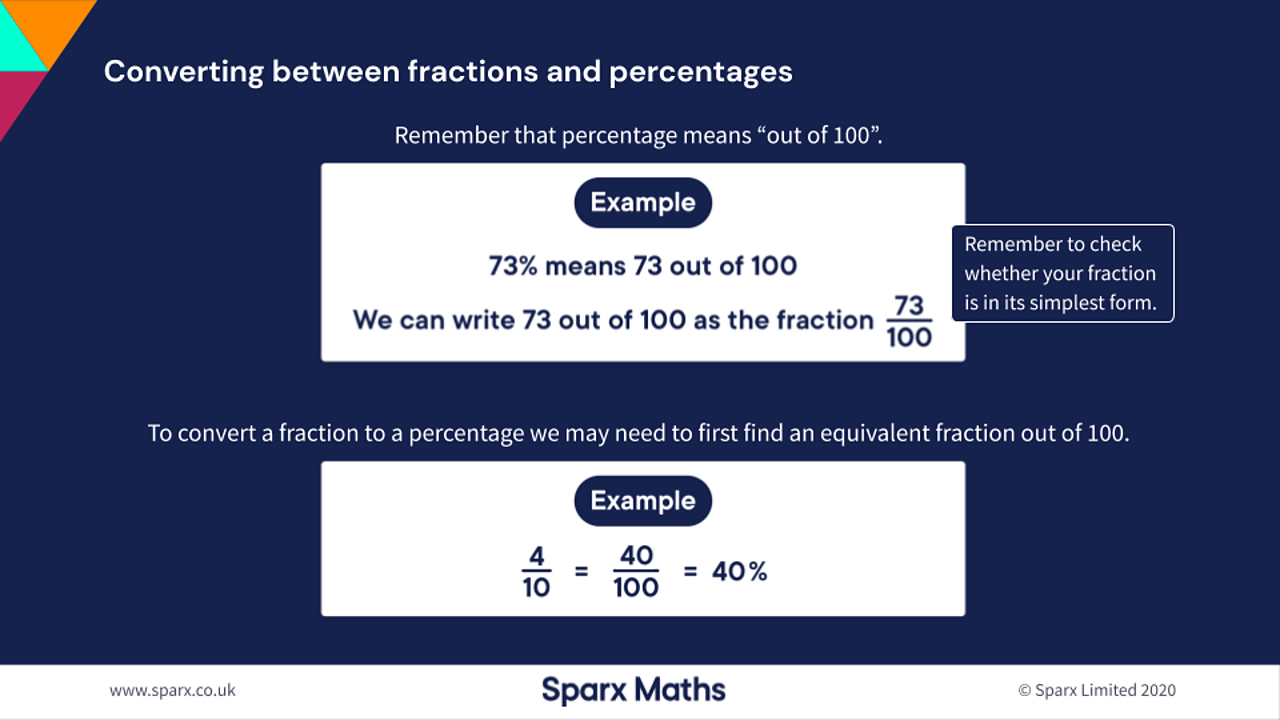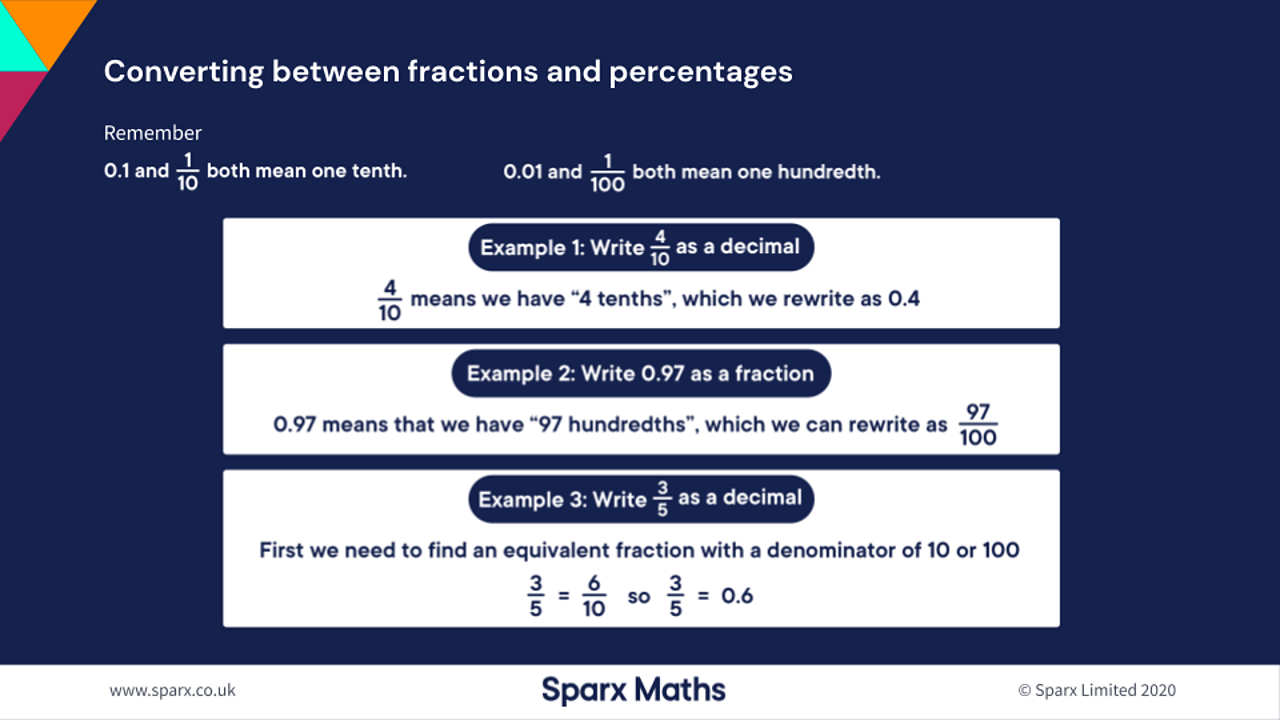# Converting fractions, decimals and percentages

## Home learning focus

In this lesson you will practice converting between simple fractions, decimals and percentages.

This lesson includes:

• a learning summary
• two activities

Created in partnership with Sparx.

# Learn

Fractions, decimals and percentages can be used to write the same number in different ways.

For example, ⁷³⁄₁₀₀, 73% and 0.73 are all ways of writing 73 parts out of 100 parts.

Some common conversions are shown on the number line below:

You won’t want to always use a number line, though. Use the slides below to learn how to convert between fractions, decimals and percentages.½ = 0.5 = 50%, ¼ = 0.25 = 25%, ¹⁄₁₀ = 0.1 = 10% and ¹⁄₁₀₀ = 0.01 = 1%.1 of 4To convert a decimal to a percentage, multiply it by 100 and write a % sign. To convert a percentage to a decimal, divide it by 100 and remove the % sign.2 of 4Percentage means 'out of 100'. You can write 73 out of 100 as the fraction ⁷³⁄₁₀₀. To convert a fraction to a percentage you may need to first find an equivalent fraction out of 100.3 of 40.1 and ¹⁄₁₀ both mean 'one tenth'. 001 and ¹⁄₁₀₀ both mean 'one hundredth'.4 of 4

Read pages 1 - 4 our 'Converting fractions, decimals and percentages' Bitesize revision guide for more examples of:

# Practise

## Activity 1

Quiz

Have a go at this quiz to find out how much you know about converting fractions, decimals and percentages!

## Activity 2

Worksheet: Fractions, decimals and percentages

Have a go at this worksheet from Sparx on converting fractions, decimals and percentages. You can print it out or write your answers on a piece of paper.

# There's more to learn

Have a look at these other resources around the BBC and the web.